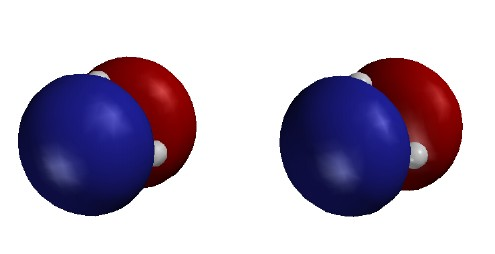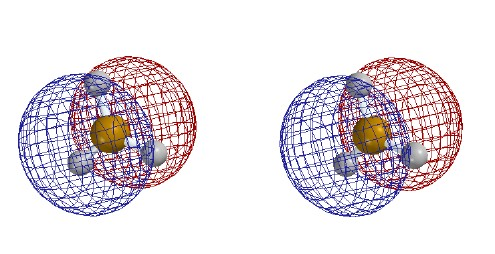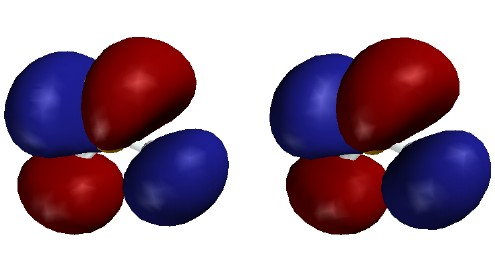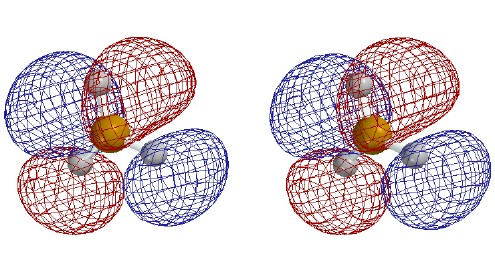BH3, an Example of an MO Computation
and an Apology for Local Bonds

Quantum mechanical calculations on smallish molecules are now routine. Although most of our thinking in Chem 125 will be based on more qualitative application of quantum mechanical ideas, it is useful to see what the results of such a calculation look like as a check on our qualitative approach to understanding bonds.

First one might consider the different goals of the various approaches to determining electron distribution in molecules:

(1) The MOLECULE does not have to deal with mathematics. It just does naturally what is necessary to achieve its GOAL: to minimize the kinetic and coulombic potential energy of its nuclei and electrons. This results in a dynamic cloud of rapidly moving electrons within which the slowly moving nuclei are embedded, rather like an "inverted" plum-pudding atom of J.J. Thomson.

Various aspects of the nature of the molecule are accessible from such experiments as:

X-ray diffraction, which measures electron density in crystals,

IR spectroscopy, which measures differences between allowed vibrational energy levels for motion of the nuclei,

ESR spectroscopy, which measures electron density at magnetic nuclei (e.g. 13C) for an "odd" electron, one whose magnetism is not cancelled by pairing with another electron.

(2) The COMPUTER has a different GOAL: to compute electron densities and molecular energy by applying some approximate version of the Schrödinger equation in order to minimize the kinetic and potential energy for the set of particles. Because the equation is too complex to solve exactly, the computer makes approximations that, hopefully, provide an acceptable compromise between affordability and realism. Typical approximations include holding the nuclei fixed while calculating the electronic wave functions (Born-Oppenheimer approximation), treating a many-electron wave function as a product of molecular orbitals, approximating the molecular orbitals as weighted sums of a limited number of atomic orbitals on different atoms, and approximating the atomic orbitals by functions that are mathematically convenient (this is the "basis set"). Programs can then correct approximately for electron-electron repulsion using SCF. More sophisticated (and computationally intensive) methods address the problem of dynamic correlation.

Computational results may be used to predict, and can be checked by, experiment. One can calculate the structure, that is the nuclear arrangement that minimizes molecular energy, or compare the energy of two different arrangements of the same atoms (before and after reaction, for example). Calculations also dissect the total electron density into individual orbitals, which suggest one-electron properties, such as the density at a magnetic nucleus (ESR), or the energy required to move an electron from one orbital to another (uv-visible light absorption), or to remove it from the molecule altogether (ionization potential).

(3) The STUDENT in Chemistry 125 has a different GOAL: to understand structure and reactivity of organic molecules. Given the limitations of the human mind, this requires finding the simplest realistic model for bonding. There is an unavoidable tension between simplicity and realism. A model of atoms with little hooks captures some of the features of bonding but it is obviously too simple to be realistic.

The realism of the model can be judged by comparing not only to experiment, but also to computation. We will not demand the quantitative precision that the computer might achieve, but the model must be quantum mechanically sound. To be worthwhile it should take us well beyond where we can go with the classical idea of lines for bonds. If it will sometimes fail, we must be aware when to expect failure.

The point of this web page is to evaluate our model of bonding by comparison with the results of a quantum mechanical computation.

The following figures were generated by "MacSpartan", a typical package that does very useful molecular orbital calculations at the SCF level. But we made no attempt to estimate correlation energy. We expect the computed electron density to be pretty faithful to nature, but there will be a errors in energy (which we hope will mostly cancel out when we do comparisons with computations for other arrangements of the same atoms).

The first series of stereo-pair pictures shows different visualizations of the TOTAL electron density of the planar BH3 molecule. It represents the sum of the electron densities calculated for the three occupied valence orbitals of this molecule and for the 1s orbital on boron. Since this computation used a reasonably large basis set of 21 atomic functions (called the "6-31G*" basis set), this total electron density is probably a very good approximation of what might be observed experimentally by x-ray diffraction, if it were possible to grow a crystal of BH3 molecules. This is of course an impossible experiment, because, as we will discuss very soon, BH3 molecules react in pairs to give B2H6. Note that the shape depends significantly on which contour is shown.Total Electron Density Note: these are stereo-pair pictures that may be viewed in three dimensions Contour level 0.30 e/Ĺ3 Electron density higher near boron than near hydrogen despite H's greater valence-shell electronegativity. This is probably due both to 1s core electrons and to the 2s electron density within the radial node. [Program not so good at drawing small surfaces - fails to draw smooth shapes and omits one H in error.]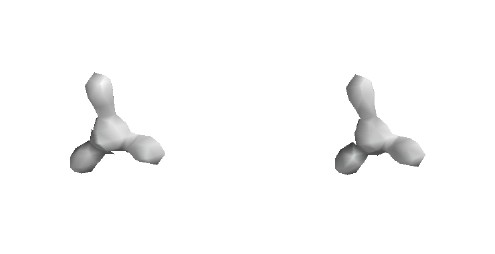Total Electron Density Contour level 0.15 e/Ĺ3 H density begins to "overtake" B density, which is high near the nucleus, but falls away quickly with increasing distance.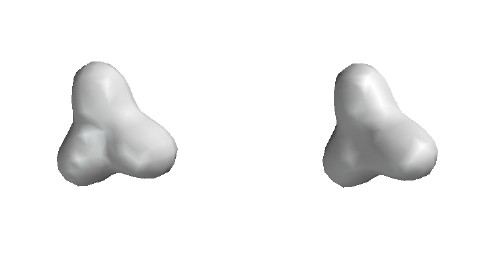Total Electron Density Contour level 0.05 e/Ĺ3 Note beginning of dimple near central B. H density is dominant for the valence electrons.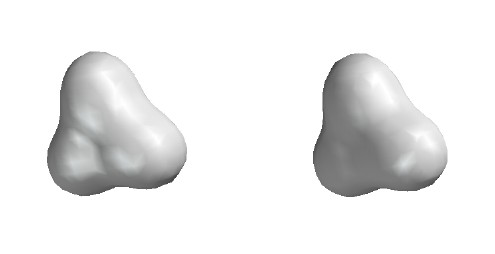Total Electron Density Contour level 0.02 e/Ĺ3Total Electron Density Contour level 0.002 e/Ĺ3 (This contour level approximates the "size" of molecules. Adjacent molecules in solids tend to be spaced such that their 0.002 surfaces touch. Thus this is a sort of "van der Waals surface".)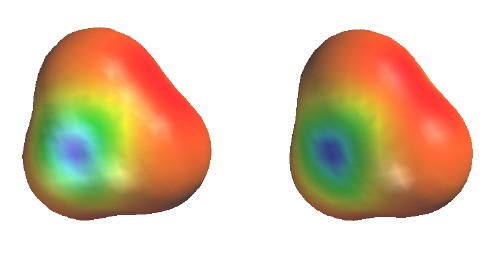Total Electron Density colored to show electrostatic potential Contour level 0.002 e/Ĺ3 (as in previous figure) Colors show the energy of a test "proton" at various positions on the surface. Red shows low energy (negative region) near H; Blue shows high energy (positive region) near B. Uneven electron distribution due to poor energy match of B-H bond (1s of H lower than 2sp2 hybrid of BTotal Electron Density with electrostatic potential Contour level 0.002 e/Ĺ3 (as in previous figure but drawn as mesh to allow seeing 0.02 contour and the ball and spoke model inside.)

The following figures show how the computer parses the total electron density into SCF molecular orbitals (presented in order of increasing energy, omitting the lowest MO which is mostly the 1s core orbital on B). Since there are 7 valence-level atomic orbitals (1s on each of three H atoms plus 2s and three 2p AOs on B) there are 7 orthogonal low-energy molecular orbitals that can be made from them. (Higher-energy MOs are constructed by mixing AOs with n > 2.) There are 6 valence electrons (i.e. three pairs: 3 electrons from B, 1 from each H), so only the first three of these valence-level MOs are occupied. Each MO is shown as two stereo pairs. The pair on the left shows the contour at a given magnitude of psi colored red or blue to denote the sign of the wave function. The pair on the right shows the surface as a mesh to reveal the ball and spoke model.Note that none of the three occupied MOs has a node between B and H. All of them contribute to B-H bonding.

For simplicity of understanding we in Chem 125 will parse the total electron density differently, into three localized and equivalent B-H bonds, rather than into a lowest energy orbital with no nodes (at the top) and two higher-energy orbitals with one node each (the two immediately above). It is not surprising that these two models predict the same overall total electron density and energy, because the nodeless MO (the analogue of atomic 2s) can be viewed as the sum of the three bonding orbitals; the first one-node MO (2px), as mostly the "vertical" B-H bond minus a lesser amount of the sum of the "horizontal" B-H bonds; and the second one-node MO (2py), as the difference between the "horizontal" B-H bonds.  Note that these MOs are bonding between B and H; their nodes pass through the B nucleus, not between B and H.

When the three localized B-H bonds are allowed by the computation to mix and form the delocalized MOs, one MO combination (the one with no nodes) goes down in energy and the other two go up, but the overall energy and electron density remains very nearly the same. Furthermore, the individual MO energies don't shift very much, because the B-H bond orbitals don't overlap well one another. Typically bond orbitals overlap less than 10% as much as the hybrid atomic orbitals whose overlap created the two bonds in the first place, so the shifts are small.

Thus, especially for qualitative purposes, we are completely justified in confining our attention to simpler localized bonding orbitals, rather than coping with their combination into the more complicated delocalized SCF MOs that the computer chooses. Since the delocalized orbitals may be viewed as a combination of the localized orbitals, the factors that influence the total energy of the localized orbitals (for example distortion from a planar toward a pyramidal structure) will influence the total energy of the delocalized MOs in the same way.

This comparison with the "true" computational orbitals justifies our use of the simpler local bond model.

When will our simplification into localized bonds fail?

(1) When (e.g. in the interaction with light) we are interested in the individual energy, or the spatial distribution, of a single pair of electrons, rather than the overall energy or total electron density.

(2) When (e.g. in the case of resonance) certain bonding orbitals have strong overlap.

(3) When (e.g. in what we will call "pericyclic" reactions) we are interested in multiple contacts between individual molecules that might lead to reactivity at more than one position.

In such cases mixing of the localized bonding orbitals can indeed make a difference, and we shall have to use a more sophisticated MO model.

The 4th MO, the LUMO of BH3, is in fact an atomic 2p orbital of B. It is like the 2p SOMO that is half-occupied by the seventh electron in the analogous CH3 radical.

Note that the last three (highest energy) UMOs have nodes between B and H. This is especially clear in the 5th MO (the 3s), which looks like a red cylinder among three blue balls. These MOs are combination of localized B-H antibonds in the same way that the lowest three MOs are combinations of B-H bonds.